Practice Test: Table Charts - 3

# Practice Test: Table Charts - 3

Test Description

## 5 Questions MCQ Test General Aptitude for GATE | Practice Test: Table Charts - 3

Practice Test: Table Charts - 3 for Banking Exams 2023 is part of General Aptitude for GATE preparation. The Practice Test: Table Charts - 3 questions and answers have been prepared according to the Banking Exams exam syllabus.The Practice Test: Table Charts - 3 MCQs are made for Banking Exams 2023 Exam. Find important definitions, questions, notes, meanings, examples, exercises, MCQs and online tests for Practice Test: Table Charts - 3 below.
Solutions of Practice Test: Table Charts - 3 questions in English are available as part of our General Aptitude for GATE for Banking Exams & Practice Test: Table Charts - 3 solutions in Hindi for General Aptitude for GATE course. Download more important topics, notes, lectures and mock test series for Banking Exams Exam by signing up for free. Attempt Practice Test: Table Charts - 3 | 5 questions in 7 minutes | Mock test for Banking Exams preparation | Free important questions MCQ to study General Aptitude for GATE for Banking Exams Exam | Download free PDF with solutions
 1 Crore+ students have signed up on EduRev. Have you?
Practice Test: Table Charts - 3 - Question 1

### Study the following table and answer the questions based on it.What is the average amount of interest per year which the company had to pay during this period?

Detailed Solution for Practice Test: Table Charts - 3 - Question 1

Average amount of interest paid by the Company during the given period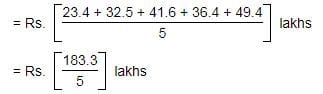= Rs. 36.66 lakhs.

Practice Test: Table Charts - 3 - Question 2

### Study the following table and answer the questions based on it.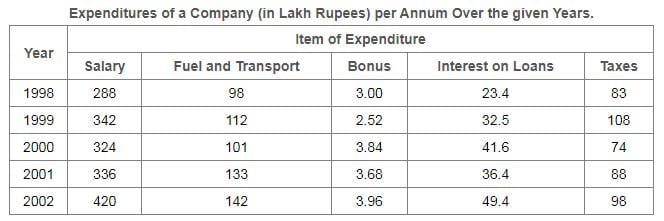The total amount of bonus paid by the company during the given period is approximately what percent of the total amount of salary paid during this period?

Detailed Solution for Practice Test: Table Charts - 3 - Question 2

[(300 + 2.52 + 3.84 + 3.68 + 3.96)*100]/[288 + 342 + 324 + 336 + 420]
= (17/1710)*100
which is approximately equal to 1.

Practice Test: Table Charts - 3 - Question 3

### Study the following table and answer the questions based on it.Total expenditure on all these items in 1998 was approximately what percent of the total expenditure in 2002?

Detailed Solution for Practice Test: Table Charts - 3 - Question 3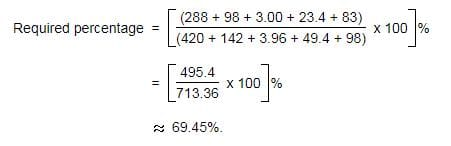Practice Test: Table Charts - 3 - Question 4

Study the following table and answer the questions based on it.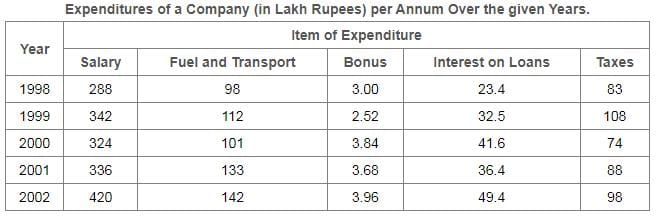The total expenditure of the company over these items during the year 2000 is?

Detailed Solution for Practice Test: Table Charts - 3 - Question 4

Total expenditure of the Company during 2000
= Rs. (324 + 101 + 3.84 + 41.6 + 74)lakhs
= Rs. 544.44 lakhs.

Practice Test: Table Charts - 3 - Question 5

Study the following table and answer the questions based on it.The ratio between the total expenditure on Taxes for all the years and the total expenditure on Fuel and Transport for all the years respectively is approximately?

Detailed Solution for Practice Test: Table Charts - 3 - Question 5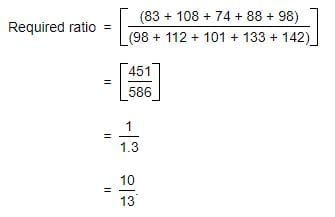## General Aptitude for GATE

73 videos|86 docs|108 tests
 Use Code STAYHOME200 and get INR 200 additional OFF Use Coupon Code
Information about Practice Test: Table Charts - 3 Page
In this test you can find the Exam questions for Practice Test: Table Charts - 3 solved & explained in the simplest way possible. Besides giving Questions and answers for Practice Test: Table Charts - 3, EduRev gives you an ample number of Online tests for practice

## General Aptitude for GATE

73 videos|86 docs|108 tests